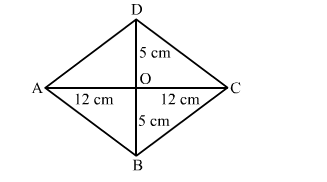# Find the length of each side of a rhombus whose diagonals are 24 cm and 10 cm long.

Question:

Find the length of each side of a rhombus whose diagonals are 24 cm and 10 cm long.

Solution:Let ABCD be the rhombus with diagonals AC and BD intersecting each other at O.
We know that the diagonals of a rhombus bisect each other at right angles.
"> If AC = 24 cm and BD =10 cm, AO = 12 cm and BO = 5 cm
Applying Pythagoras theorem in right-angled triangle AOB, we get:

$A B^{2}=A O^{2}+B O^{2}=12^{2}+5^{2}=144+25=169$

$A B=13 \mathrm{~cm}$

Hence, the length of each side of the given rhombus is 13 cm.